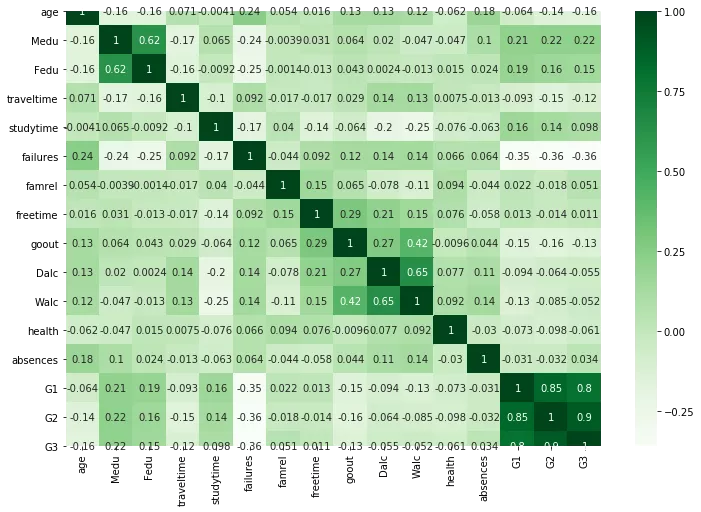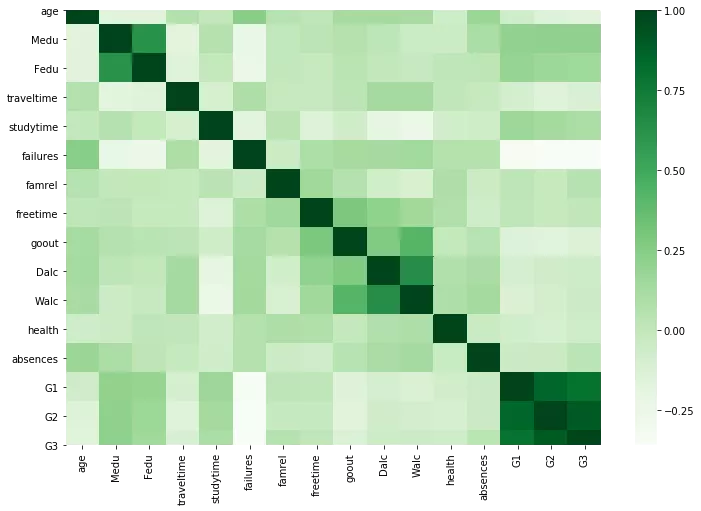# How To Use Pandas Correlation Matrix

Correlation martix gives us correlation of each variable with each of other variables present in the dataframe. To calculate correlation, we first calculate the covariance between two variables and then covariance is divided by the product of standard deviation of same two variables. Correlation has no units so it is easy to compare correlation coeffient.

In pandas, we dont need to calculate co-variance and standard deviations separately. It has corr() method which can calulate the correlation matrix for us.

If we run just df.corr() method. We would get correlation matrix for all the numerical data.

Let us first import the necessary packages and read our data in to dataframe.

In :
import pandas as pd

In :
from matplotlib import pyplot as plt


I will use students alcohol data which I downloaded from following UCI website...

archive.ics.uci.edu/ml/datasets/student+performance

In :
df = pd.read_csv('student-mat.csv')

In :
df.head(2)

Out:
school sex age address famsize Pstatus Medu Fedu Mjob Fjob ... famrel freetime goout Dalc Walc health absences G1 G2 G3
0 GP F 18 U GT3 A 4 4 at_home teacher ... 4 3 4 1 1 3 6 5 6 6
1 GP F 17 U GT3 T 1 1 at_home other ... 5 3 3 1 1 3 4 5 5 6

2 rows × 33 columns

Most of the variables are self explanatory except the following ones...

• G1 - first period grade (numeric: from 0 to 20)
• G2 - second period grade (numeric: from 0 to 20)
• G3 - final grade (numeric: from 0 to 20, output target)
• Mjob - Mothers Job
• Fjob - Fathers Job

In :
corr = df.corr()


For too many variables, correlation matrix would be pretty big. Therefore it is best to visualize the correlation matrix.

To visualize we can use seaborn library.

In :
import seaborn as sns

In :
plt.figure(figsize=(12,8))
sns.heatmap(corr, cmap="Greens",annot=True)

Out:
<matplotlib.axes._subplots.AxesSubplot at 0x7f54f18a0810>We can ignore the diagonal values, since that is correlation of variable with itself.

values to the left and right of diagonal are mirror image of each other. The greater the correlation between variables, the darker the box is. Therefore we dont need to print the value in each box, since it makes our heatmap ugly. We can look at the color of the box to conclude which are the variables with high correlation.

In :
plt.figure(figsize=(12,8))
sns.heatmap(corr, cmap="Greens")

Out:
<matplotlib.axes._subplots.AxesSubplot at 0x7f54ec3a8a90>In case you need to print the values of correlation matrix in the descending order. use sort_values() to do that as shown below.

In :
c1 = corr.abs().unstack()
c1.sort_values(ascending = False)

Out:
G3          G3            1.000000
G2          G2            1.000000
Medu        Medu          1.000000
Fedu        Fedu          1.000000
traveltime  traveltime    1.000000
...
famrel      Medu          0.003914
Fedu        Dalc          0.002386
Dalc        Fedu          0.002386
Fedu        famrel        0.001370
famrel      Fedu          0.001370
Length: 256, dtype: float64

Ofcourse it doesnt make sense to print the diagonal values since they will be 1 any way. Let us just filter out the diagonal values.

In :
corr[corr < 1].unstack().transpose()\
.sort_values( ascending=False)\
.drop_duplicates()

Out:
G3        G2           0.904868
G1        G2           0.852118
G3           0.801468
Dalc      Walc         0.647544
Fedu      Medu         0.623455
...
Walc      studytime   -0.253785
failures  G1          -0.354718
G2          -0.355896
G3          -0.360415
age       age               NaN
Length: 121, dtype: float64

From above we can conclude that G3 and G2, G1 and G2, G1 and G3, Dalc and Walc are highly correlated variables.